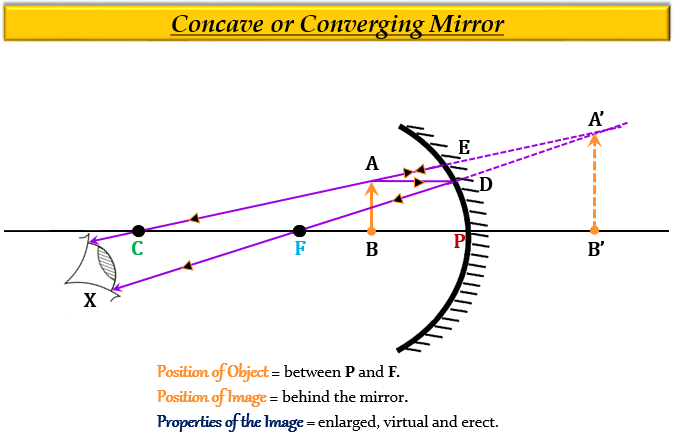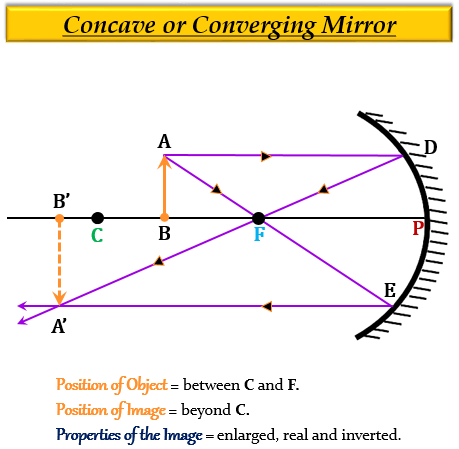# (a) Give two circumstances in which a concave mirror can form a magnified image of an object placed in front of it. Illustrate your answer by drawing labelled ray diagrams for both.(b) Which one of these circumstances enables a concave mirror to be used as a shaving mirror?

(a) The two circumstances in which a concave mirror can form a magnified image of an object that is placed in front of it are:

1. When an object is placed between the pole $(P)$ and the focus $(F)$ of a concave mirror, the image formed is virtual, erect and magnified, as shown in the figure given below. Here, the object $AB$ is placed between the pole $(P)$ and the focus $(F)$ of a concave mirror, and its image $A'B'$ is formed behind the mirror which is virtual, erect and magnified.2. When an object is placed between the centre of curvature $(C)$ and the focus $(F)$ of a concave mirror, the image formed is real, inverted and enlarged, as shown in the figure given below. Here, the object $AB$ is placed between the centre of curvature $(C)$ and the focus $(F)$ of a concave mirror, and its image $A'B'$ is formed beyond the centre of curvature which is real, inverted and enlarged.(b) From the above-mentioned circumstances, only first circumstances, where the object is placed between the pole $(P)$ and the focus $(F)$ of a concave mirror, can be used as a shaving mirror, because, the image formed is virtual, enlarged and erect.

Updated on: 10-Oct-2022

51 Views